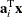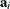# The OPTQP Procedure

### Output Data Sets

This section describes the PRIMALOUT= and DUALOUT= output data sets. If the SAVE_ONLY_IF_OPTIMAL option is not specified, the output data sets do not contain an intermediate solution.

#### Definitions of Variables in the PRIMALOUT= Data Set

The PRIMALOUT= data set contains the primal solution to the quadratic programming (QP) model. The variables in the data set have the following names and meanings.

_OBJ_ID_

specifies the name of the objective function. Naming objective functions is particularly useful when there are multiple objective functions, in which case each objective function has a unique name. See the section ROWS Section for details.

Note: PROC OPTQP does not support simultaneous optimization of multiple objective functions in this release.

_RHS_ID_

specifies the name of the variable that contains the right-hand-side value of each constraint. See the section ROWS Section for details.

_VAR_

specifies the name of the decision variable.

_TYPE_

specifies the type of the decision variable. _TYPE_ can take one of the following values:

N

nonnegative variable

D

bounded variable with either lower or upper bound

F

free variable

X

fixed variable

O

other

_OBJCOEF_

specifies the coefficient of the decision variable in the linear component of the objective function.

_LBOUND_

specifies the lower bound on the decision variable.

_UBOUND_

specifies the upper bound on the decision variable.

_VALUE_

specifies the value of the decision variable.

_STATUS_

specifies the status of the decision variable. _STATUS_ can indicate one of the following two cases:

O

The QP problem is optimal.

I

The QP problem could be infeasible or unbounded, or PROC OPTQP was not able to solve the problem.

#### Definitions of Variables in the DUALOUT= Data Set

The DUALOUT= data set contains the dual solution to the QP model. Information about the objective rows of the QP problems is not included. The variables in the data set have the following names and meanings.

_OBJ_ID_

specifies the name of the objective function. Naming objective functions is particularly useful when there are multiple objective functions, in which case each objective function has a unique name. See the section ROWS Section for details.

Note: PROC OPTQP does not support simultaneous optimization of multiple objective functions in this release.

_RHS_ID_

specifies the name of the variable that contains the right-hand-side value of each constraint. See the section ROWS Section for details.

_ROW_

specifies the name of the constraint. See the section ROWS Section for details.

_TYPE_

specifies the type of the constraint. _TYPE_ can take one of the following values:

L

less than or equals constraint

E

equality constraint

G

greater than or equals constraint

R

ranged constraint (both less than or equals and greater than or equals)

See the sections ROWS Section and RANGES Section (Optional) for details.

_RHS_

specifies the value of the right-hand side of the constraints. It takes a missing value for a ranged constraint.

_L_RHS_

specifies the lower bound of a ranged constraint. It takes a missing value for a non-ranged constraint.

_U_RHS_

specifies the upper bound of a ranged constraint. It takes a missing value for a non-ranged constraint.

_VALUE_

specifies the value of the dual variable associated with the constraint.

_STATUS_

specifies the status of the constraint. _STATUS_ can indicate one of the following two cases:

O

The QP problem is optimal.

I

The QP problem could be infeasible or unbounded, or PROC OPTQP was not able to solve the problem.

_ACTIVITY_

specifies the value of a constraint. In other words, the value of _ACTIVITY_ for the ith constraint is equal to, whererefers to the ith row of the constraints matrix anddenotes the vector of current decision variable values.